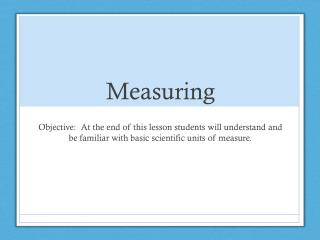DownloadDownload PresentationMeasuring

# Measuring

Télécharger la présentation## Measuring

- - - - - - - - - - - - - - - - - - - - - - - - - - - E N D - - - - - - - - - - - - - - - - - - - - - - - - - - -
##### Presentation Transcript

1. Measuring Objective: At the end of this lesson students will understand and be familiar with basic scientific units of measure.

2. Using a Metric Ruler • In science a metric ruler is used. The units on a metric ruler are called centimeters (cm) and millimeters(mm).

3. The Marks on a Ruler • Each of the long marks on a metric ruler represents one centimeter (cm). • Each of the short marks on a metric ruler represents one millimeter (mm). • There are 10 small marks or millimeters (mm) in every centimeter (cm).

4. How long are the following lines in millimeters and centimeters? 0.8 8 1.1 11 0.4 4

5. Liquid Measure • Scientists use liters (L) and milliliters (mL) to measure liquids. • 1000 mL is equal to 1 L

6. Taking Accurate Liquid Measurements • When measuring a liquid you must measure at the base of the meniscus.

7. Dry Measure • Scientists measure dry material in grams (g) and milligrams (mg). • 1000 mg is equal to 1g

8. Temperature • Scientists use the Celsius or Kelvin scale to measure temperature.